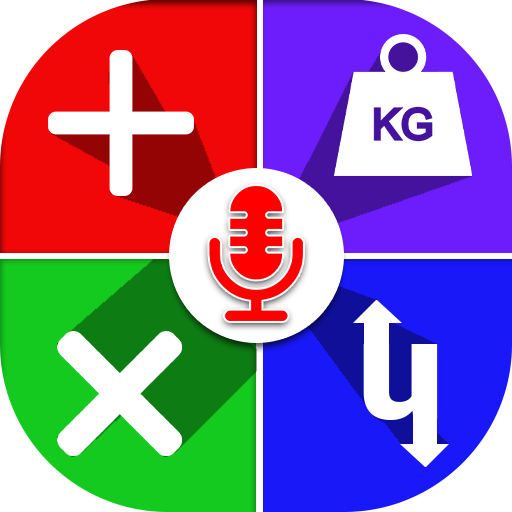## Voice Calculator & Unit Converter 2020

### by Digital Trend

Free
922
Categories Tags
###### Description

Voice Calculator 2020- Online Scientific & Unit Converter Calculator

Multiply 2020 is a voice calculator & unit conversion app where you can do fast calculations by voice.

This App contains lots of categories where you can do Trigonometry & multi division calculations by using our online & offline voice calculator.

Multiply is an amazing app for android phones. In our daily life, a calculator is the basic need of every person. Most students use a school calculator but college & university students mostly use a scientific calculator so on the very demand of our users we launched a free voice calculator online app on the play store.

In multiply, you can do all types of calculations & conversations like an inch to cm, etc by using our All in one calculator.

Multiply supports the various calculations like Division Calc smart computation unit conversion trigonometry Calculation

It calculates as per DMAS rule and gives accurate answers. It also supports percentage calculation. For quick scientific calculation of Sin Cos and Tan, degrees use to multiply it works fast.

how to use this Online Voice Calculator & Unit Converter App?

You have to use our Online calc app because in this App, solve the complicated calculation just speak in front of mick, you will get the result in text form. Most users use a calculator for scientific calculation so our app contains all categories.

How to use Multiply 2020?

Open calculator & first read Instruction
Just click on a mick button and enter your values by talk calculator
For Addition: Just speak Two plus Two or 2+2 result Will be on your screen
For long Expression: Just speak One plus two minus three divided by two or 1+2-3/2 You will get the answer & result of your values in seconds
Add new value to the previous result
Clear all result by clicking the clear button
For unit, conversion go to conversion fragment
Inch to cm or pounds to kg converter

if you want to exit click on phone back button & press exit
Don't forget your feedback rate us out of 5

Features of Free Voice Calculator Online 2020:

Voice Detector
Unit converter
Trigonometry Calculator
Multiply supports in the English language.
Auto calculate your division with other expressions
Easily use scientific calculator & factorial
You can easily find values of sin, cos, tan & log
Solve Square root value & power calculation
Unit conversion calculator & calculation by voice
Expressions & percentage
The calculator will give you a very accurate answer by Continuing with the previous function answer.
By giving instruction to an old answer get a new result
Inches to cm and mm converter

Support Languages: Standard language (English)

Unit conversion and Smart calculator
Voice Scientific Calculator
Trigonometry & Fast calculator
All in one Calculator
Pounds to kg or kg to grams converter

Units:
inches, cm, mm, pounds, kg, grams & ounces you can do the conversion of all these mention units.

Voice Calculator & Unit Conversion is special because our all in one calculator work on the user's voice.

Note:
It only supports the standard English language. If you like our voice calculator & Unit converter 2020 app, then give your feedback in the review section or for more features keeps using the best calculator 2020 App.

Thank you.

110

1038
• AOTD

2517